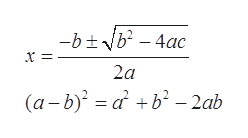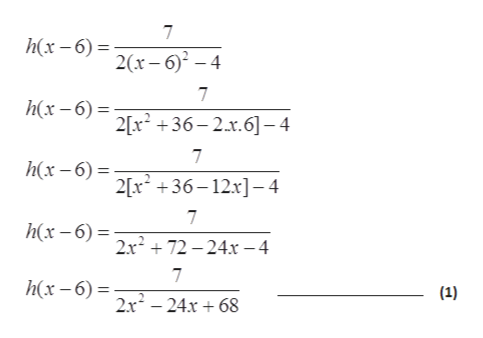GRAPHS AND FUNCTIONSVariable expressions as inputs of functions: Problem type 27The function h is defined as h (x)=22x-4Find h (x-6)Write your answer without parentheses, and simplify it as much as possible.h (x-6)=미□미X

Question

See attachedhelp_outlineImage TranscriptioncloseGRAPHS AND FUNCTIONS Variable expressions as inputs of functions: Problem type 2 7 The function h is defined as h (x)= 2 2x-4 Find h (x-6) Write your answer without parentheses, and simplify it as much as possible. h (x-6) = 미□미 X fullscreen
Step 1

The function h is defined as:

Step 2

Formula used:

Roots of the quadratic equation:help_outlineImage Transcriptioncloseー6士V-4ac x = 2a (a-b) a b-2ab fullscreen
Step 3

Simply the value of ...help_outlineImage Transcriptionclose7 h(x-6) 2r-6)-4 7 h(x-6)2x +36-2.x.6]-4 7 h(x-6) 2[x3612x-4 7 h(x-6)2x + 72 - 24x -4 7 h(x-6) (1) 2x224x68 fullscreen

Want to see the full answer?

See Solution

Want to see this answer and more?

Our solutions are written by experts, many with advanced degrees, and available 24/7

See Solution
Tagged in

Other